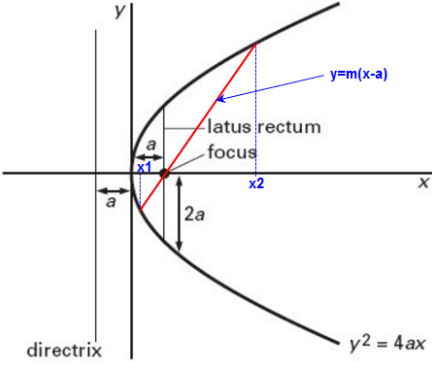Q

# Solve this problem - Let A(4,-4) and B(9,6) be points on the parabola,. Let C be chosen on the arc AOB of the parabola, - Co-ordinate geometry - JEE Main

Let A(4,-4) and B(9,6) be points on the parabola, . Let C be chosen on the arc AOB of the parabola, where O is the origin,such that the area of  is maximum. Then, the area (in sq. units) of  is

• Option 1)

• Option 2)

• Option 3)

• Option 4)

32

Views

Standard equation of parabola -

- whereinParabolaAny point on the parabola is

Here,

SO,

Aera

Aera is maximum when

Option 1)

Option 2)

Option 3)

Option 4)

32

Exams
Articles
Questions